Number Knowledge and Error Types of Elementary Portuguese Students: Implications for Instruction | OMICS International
Clinical and Experimental Psychology

Like us on:

# Number Knowledge and Error Types of Elementary Portuguese Students: Implications for Instruction

Silvana MR Watson1*, Sharon Judge1, João Lopes2, Célia Oliveira3 and Ana Catarina Jesus2

1Old Dominion University, Norfolk, VA, USA

2University of Minho, Braga, Portugal

3Lusófona University of Porto, Porto, Portugal

*Corresponding Author:
Watson MR
Old Dominion University, Norfolk, VA, USA
Tel: + (757) 683-6364
E-mail: [email protected]

Received Date: January 02, 2016; Accepted Date: January 09, 2016; Published Date: January 16, 2016

Citation: Watson SMR, Judge S, Lopes J, Oliveira C, Jesus AC (2016) Number Knowledge and Error Types of Elementary Portuguese Students:Implications for Instruction. Clin Exp Psychol 2: 110. doi: 10.4172/2471-2701.1000110

Copyright: © 2016 Watson SMR, et al. This is an open-access article distributed under the terms of the Creative Commons Attribution License, which permits unrestricted use, distribution, and reproduction in any medium, provided the original author and source are credited.

Visit for more related articles at Clinical and Experimental Psychology

#### Abstract

In the present study, we examined number knowledge skills of 697 Portuguese elementary students from first to fourth grade. Students completed three number knowledge tasks: 1) translating numbers into words, 2) symbolic magnitude (i.e., number comparison), and 3) decomposing numbers. We evaluated students’ answers by means of error analysis using a three-category coding system adopted from specific error types were computed by grade level. Results showed that there were significant differences among grades and that the prerequisite linguistic error type (i.e., pre linguistic rules or principles of the cardinal number system), particularly in the magnitude tasks, significantly contributed to students’ performance on number knowledge tasks. This is important for instruction because learning difficulties in mathematics have been associated with weaknesses in intermediate number knowledge competencies (e.g., number comparison). Our qualitative data analyses suggest that instruction, intervention, or remediation need to consider systematic instructing students the prelinguistic rules of the number system, specifically the principles related to larger numbers.

#### Keywords

Mathematics; Number knowledge; Error analysis; Intervention

#### Introduction

Student mathematical skills normally are acquired through a cumulative and progressive learning process. Numeracy or number knowledge (i.e., the understanding of whole numbers and number relationships) has been identified as a predictor of successful mathematics learning [1-6]. Most children are able to acquire number knowledge skills when taught informally (e.g., home) or formally (e.g., school) . However, not all children acquire numeracy skills easily. Theories of number competency have been linked to several factors such as family income e.g., , working memory capacity e.g., , and language abilities . Other studies have connected mathematics learning disability (MLD) to specific number knowledge tasks such as comparing and naming digits [11-13]. Researchers have found that students who have MLD are less efficient and slower in processing symbolic number tasks such as magnitude comparison tasks (i.e., which number is larger?) than typically developing children [14,15].

Number knowledge involves quantitative knowledge, number word knowledge, numerical relationships, estimation and the ability to effectively use numeracy in everyday life [16,17]. Errors in number knowledge tasks reveal a student’s difficulties in one or more types of knowledge. Students may not have the vocabulary to produce number words, the knowledge of the rules to produce the correct numbers according to their location, or know the conventions of the cardinal number system (e.g., organize digits in groups of three and use commas). If errors are persistent and systematic, they can provide information on what a student did not understand (i.e., conceptual knowledge) or did not master (i.e., procedural knowledge; [18,19]. Error analysis of numerical competence provides a diagnosis of a student’s learning and areas of number competence which is critical to developing a plan of intervention remediation.

Some researchers have examined the types of errors children make on numeracy tasks [20,21] analyzed errors children made in changing number words into numerals. Although their studies involved a small number of participants, they observed that children had problems with the syntactic structure of number words because they added numbers, most often zeros, to the numeral they were translating from number words. On the other hand  performed an error analysis of children’s number naming. Their study included 95 students from grades one, three, five, and seven  developed an error coding system to determine the location (e.g., decades, hundreds, millions) and categorization of the types of errors children make in orally producing numbers in words. They based their coding system on several cognitive models and research [22-25]. Errors were classified according to three categories: 1) prerequisite linguistic (i.e., prelinguistic rules or principles of the cardinal number system for converting numbers into words, 2) vocabulary (i.e., knowledge of number words and 3) compound construction (i.e., a set of compound rules to sequence correctly the words within a number compound). The researchers found that students, in particular older students, had deficits in their knowledge of prerequisite linguistic conventions when producing larger number names.

Another group of researchers , examined the error types of 325 students between the ages of 7 and 9 years in number writing. Their study involved students with and without MLD. Similar to the results of previous studies,  found that students’ most common errors involved syntactic lexical errors. They noticed that these types of errors were more frequently made by students with MLD and low achieving students than students with average or above average mathematics ability. The ability to correctly produce number words orally or in written language (and especially larger numbers) from their arabic form in the correct sequence may influence many other mathematical skills such as counting, place value, and decimals .

Like translating numbers into words, numerical magnitude tasks often are used to measure number knowledge skill. Numerical magnitude refers to the ability to understand, estimate, sequence, and compare numbers by their sizes . Examined the relationship between symbolic and non-symbolic magnitude and overall mathematics achievement in 53 students in the fifth graders. They used numerical magnitude comparison and number line estimation tasks to measure the students’ understanding of numerical magnitude. The results of the study of  showed that both symbolic and non-symbolic magnitude understanding were related to students’ achievement in mathematics. However, the relation to mathematical learning was much stronger for symbolic numbers.

Early number system knowledge has been related to successful mathematics skills development [27,28] In fact, in a longitudinal study  found that knowledge of the number system in the first grade predicted mathematics achievement and functional numeracy skills in seventh graders (β = 0.195, p = 0.0014). One of the tasks they used to measure number knowledge was number line estimation  described number knowledge as the understanding of number magnitude, the ordering of numbers according to their size, and the ability to combine and decompose numbers into smaller or larger numbers. Jordan et al.  reported similar findings on their longitudinal study. They investigated the developmental predictors (e.g., attention, understanding of number magnitude) of the acquisition of fraction concepts and procedures. Their results supported the importance of numerical magnitude understanding to learning mathematics. Finally, they reported that number line estimation was the largest independent contributor to the acquisition of conceptual and procedural knowledge of fractions.

It has become increasingly clear that number knowledge is critical for mathematics achievement. Lack of mathematical literacy skills can seriously limit an individual’s opportunities to succeed in life [30,31]. Not surprisingly, competence in mathematics is a major concern in the United States and other countries like Portugal because a significant percentage of K-12 students are functioning below proficiency levels in mathematics [30,32]. The most recent assessment results from the Program for International Student Assessment from 2012  show the mathematics average scores for Portugal students was 487 and for the U. S. students as 481. The 2011 Trends in International of Mathematics and Science Study showed similar average scores; American 4th graders’ average score was 541 and Portuguese 4th grades’ average score was 532 .

Given the fact that American and Portuguese elementary students perform similarly in mathematics, examining the mathematics skills of Portuguese students can contribute to our understanding of typical and atypical development of elementary students’ mathematical skills. Recognizing that persistent errors in number knowledge tasks may imply incorrect or incomplete number concepts, we examined the type of errors Portuguese students made in three number knowledge tasks. We propose that a qualitative error analysis of students’ responses can provide more insight regarding the nature of students’ problem solving than just marking correct/incorrect responses. Identifying students’ type of deficiencies in number knowledge can provide educators critical information for effective intervention or remedial instruction.

The purpose of the present study was threefold: 1) to examine the type of errors Portuguese elementary students make in number knowledge tasks, 2) to identify the most common type of errors in number knowledge by grade level, and 3) to identify the type of errors that significantly contribute to student performance on number knowledge. We contend that answers to these questions should provide educators a fuller understanding of the persistent difficulties some students encounter as they progress through the mathematics curriculum.

#### Method

Participants

Participants were 697 students from 42 classrooms of seven elementary schools in northern Portugal. Students had completed the school year as first through fourth graders (Table 1). Sixty- two percent of participants came from schools where 31% of students received free lunch. The other participants (38%) were from lower socio-economic schools with 35%-51% of students receiving free lunch. There were 339 (48.6%) girls and 358 (51.6%) boys from ages 6 to 13 years old (M = 8.78 years). See Table 1 for student information. All students were receiving instruction in the general education curriculum for their particular grade level. In Portugal, learning disability is not one of the recognized disability categories. This means that students who may have a learning disability in mathematics have not been identified as having MLD. Even so, some schools provide extra help to those students struggling in mathematics.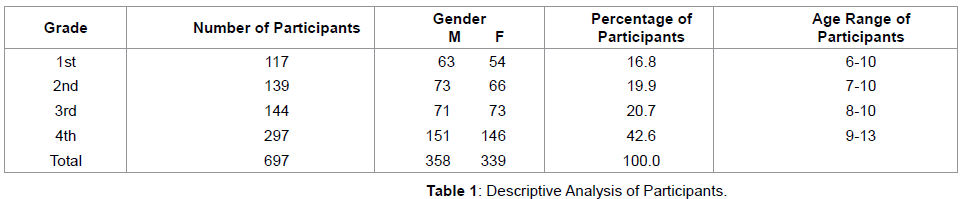#### Materials

Each student completed a written test of mathematical knowledge developed by . The instrument reflects the Portuguese mathematics curriculum of 1st through 4th grade. It is divided into three main sections: number knowledge, calculation, and problem solving. The instrument has a total of 46 items. In this article, we report on the items of the first part of the test (i.e., number knowledge) that involve numeracy tasks such as number word knowledge, magnitude comparison, and decomposing numbers. A principal component analysis, based on a tetracoric correlation matrix, revealed an initial six-factor solution for the test. A subsequent principal component analysis with an oblique rotation was conducted to test the hypothesis of a second order factor related to the math general achievement. This analysis revealed 42- item solution representing five primary factors that cluster in a single second-order factor related to general math achievement was found. The Cronbach Alpha for the general factor was 94. A psychometric analysis based on Rasch’s Model of the Item Response Theory showed that the items covered the full extension of the subjects’ skills.

#### Procedures

Students were asked to complete three number knowledge tasks: 1) Translate in writing a random set of numerals into number words; 2) Identify the biggest and smallest number within a random set of numerals (i.e., magnitude); and 3) Decompose numbers according to their place value. A three-category coding system was adopted from  who examined the developmental differences in children’s number naming errors by identifying the nature of the error and its location. The three categories were: 1) prerequisite linguistic (e.g., write digits according to value [four, five, six for 456]), 2) vocabulary (e.g., two, one hundred one for 2,101), and 3) compound construction (e.g., inconsistent syntactic structure [one thousand hundred for 100,000]). See Table 2 for the description of the three error categories. Errors were only counted when the student made the error answering his/her grade level type of problem. We also noticed when a student made more than one type of error in the same problem.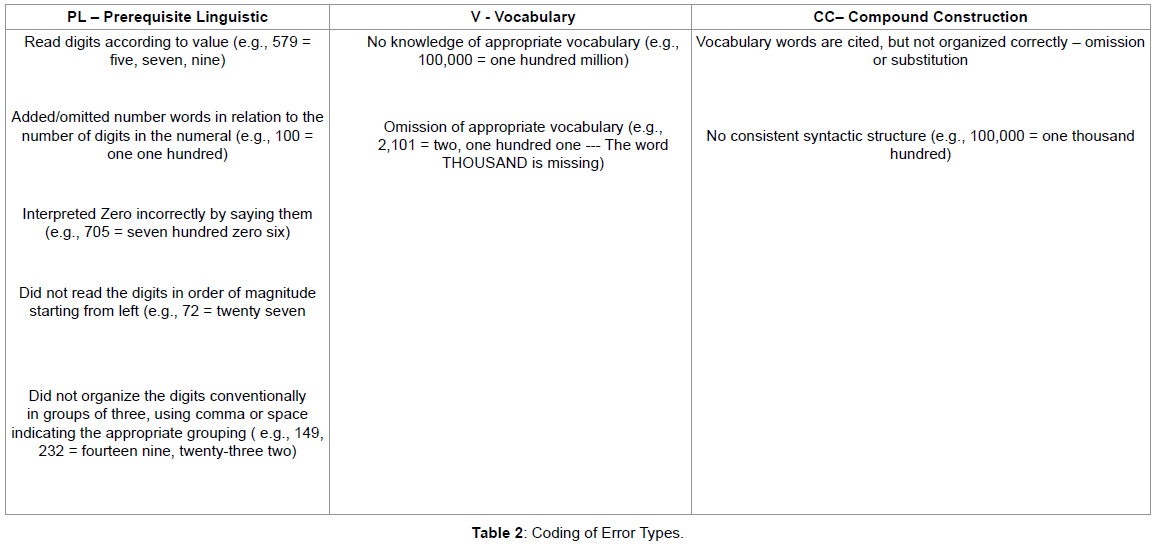Two researchers graded all tests three times. The first time, they identified correct/incorrect items. The second time, they used a code system to identify the types of errors (e.g., prerequisite linguistic error) students made. After grading all tests, a reliability check was performed on 65% (n = 456) randomly selected exams from different grade levels. Inter-observer agreement was calculated by reporting agreements on occurrences divided by agreements plus disagreement (A/ [A+D]). The percent of agreement was 0.98. The few disagreements between raters according to error type were resolved by one of the other two researchers.

#### Results

This study investigated the types of errors Portuguese elementary students make when performing three number knowledge tasks: 1) translating numbers into words, 2) magnitude comparison, and 3) decomposing numbers. Data frequency analyses showed that the most common type of errors among all 697 students were the prerequisite linguistic type (n = 863; 49.5%) and the compound construction type (n = 781; 45%). Seventy-two percent (72%) of errors in the Translate Numbers Task were the prerequisite linguistic type (n = 433). In the Magnitude Task, 99.5% of errors were the prerequisite linguistic type (n = 428). Often, students did not identify the digits in order of magnitude starting from left and/or did not organize the digits conventionally in groups of three. Several children had difficulty with numbers containing zeros (e.g., 35,026) and named them incorrectly. The compound construction error type was the most common in the Decompose Number Task (n = 710; 99%). Students had difficulty organizing the numbers according to their place value, indicating a lack of knowledge of the syntactic structure of numbers.

A Pearson’s product- moment correlation was computed to assess the relationship between grade level and error types. Preliminary analyses showed the relationship to be linear with variables normally distributed, as assessed by Shapiro-Wilk test (p>0.05), and there were no outliers. Correlation analyses indicated there was a significant positive correlation between grade level and prerequisite linguistic errors in the Translate Numbers Task, r (696) = 0.55, p <0.001, and in the Magnitude Task, r (696) = 0.21, p < 0.001. There also was a significant positive correlation between grade level and compound construct error types in the Decompose Numbers Task, r (696) = 0.34, p < 0.001. Additionally, there was a significant positive correlation between the prerequisite linguistic errors in the Translate Numbers Task and the Magnitude Task, r (696) = .25, p < .001, and compound construct error types in the Decompose Numbers Task, r (696) = 0.30, p < 0.001.

The most predominant errors at each grade level

We used frequency to depict the number of times each error type occurred at each grade level. As shown on Table 3, the most common type of errors in the task of Translating Numbers into Words was the prerequisite linguistic type in grades 3 and 4, but vocabulary error type in grades 1 and 2. In the Magnitude Tasks, prerequisite linguistic error types were the predominant errors in all four grade levels. In the Decomposing Numbers Tasks, the compound construction error types were the most common error in all four grade levels.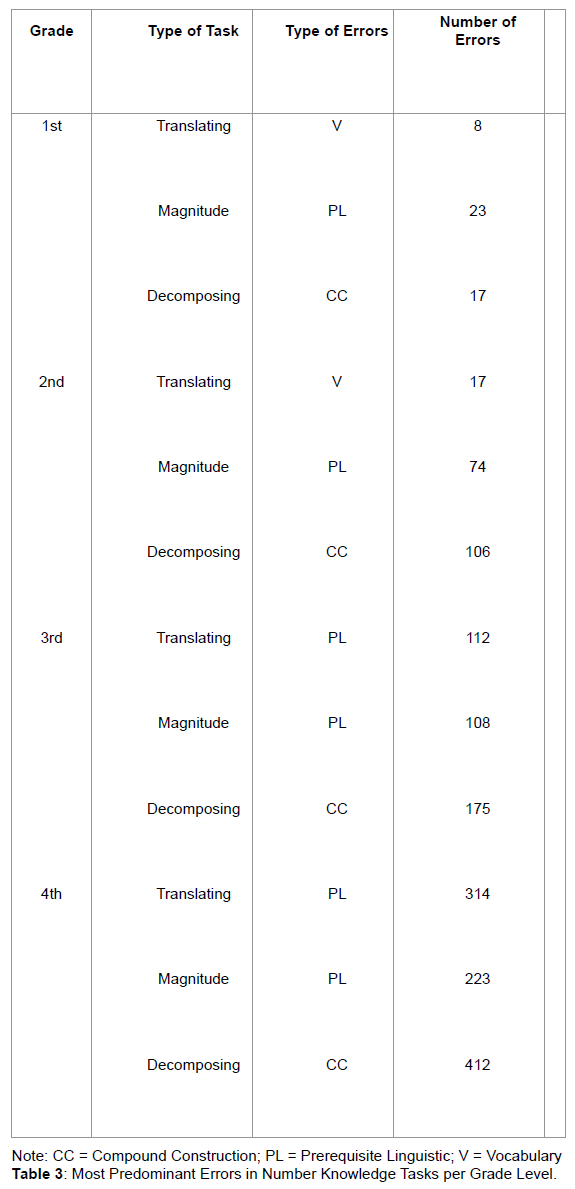Predictors of student performance

We conducted a hierarchical multiple regression analysis to determine whether each independent variable (i.e., grade level and the three most common type of errors) play a particular important role in explaining the variance of student performance in number knowledge. In the analysis, grade level of each student was entered into the regression equation first as a control variable, followed by the three most common types of number knowledge errors. This model was used to predict the total student performance in number knowledge. Inter correlations of predictor and outcome variables are presented in Table 4. This methodological approach allowed us to isolate of the relative contributions of the grade level first, followed by the individual contributions of each of the effects of number knowledge error pattern variables on the number knowledge measure . In the analysis, the increments (I) in R2 were determined to assess whether the different independent measures accounted for a significant proportion of variance in the dependent measure.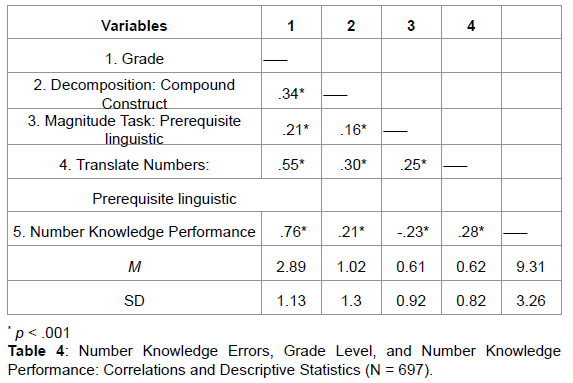As can be seen in Table 5, the result of the hierarchical regression analysis was significant (F = 468.44, p < 0.001). The addition of each predictor variable led to a statistically significant increase in R2. After controlling for the grade level variable, all three error patterns accounted for a statistically significant 16% of the variance in the number knowledge performance score. The prerequisite linguistic errors in the Magnitude Task was the strongest statistical predictor for number knowledge performance, ΔR2 = 0.15, ΔF (1,693) = 371.30, p < 0.001. The compound construct error types in the Decompose Number Task and the prerequisite linguistic errors in the Translate Numbers Task both accounted for 1% of the variance in number knowledge performance.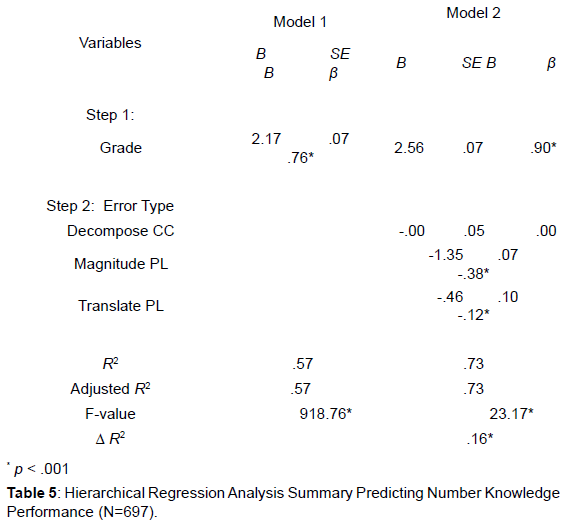#### Discussion

Number knowledge is considered to be a powerful predictor of later mathematics outcomes [2,39] and more specifically, the ability to process and discriminate between symbolic magnitudes [40-42]. The results of our study mirror the current literature and underscore the importance of numerical magnitude tasks in predicting individual student performance . Hierarchical regression analysis indicated that grade level and prerequisite linguistic knowledge of numbers in Magnitude Tasks were significantly related to students’ overall number knowledge performance. Our findings are consistent with other studies that found symbolic magnitude understanding related to mathematics performance [44-42].

Similar to the findings of [1,26,46,47] our results indicated that Portuguese students in elementary grades committed more prerequisite linguistic errors than vocabulary type errors in Translating Numbers Tasks. Students’ prerequisite linguistic errors involved reading digits without considering their place value, ignoring order magnitude from left to right, misinterpreting zeroes and commas, and adding or omitting number words in relation to the number of digits in the numeral. These findings suggest that students’ knowledge of the syntactic framework of numbers may adversely affect their ability to solve magnitude and decomposing numbers tasks. As children advance in grade levels, they are required to learn about larger numbers (e.g., thousands, millions, billions). However, if students do not have the prerequisite linguistic knowledge of numbers, they may have difficulty completing magnitude and decomposing tasks. Prerequisite linguistic knowledge of numbers can influence other error types and it may affect the processing of students’ knowledge of number skills.

#### Conclusion

By examining Portuguese elementary students’ performance on number knowledge tasks, we were able to identify students’ error patterns. The results of the studies previously discussed and our findings suggest that the understanding of magnitude numerals, the ability to orderly name numbers, and combine and decompose them into larger or smaller numbers are prerequisite to other mathematical concepts and skills. Because learning difficulties in mathematics have been associated with weak number knowledge skills such as understanding and processing numerical magnitude [11,13,48]. Our findings suggest that students in the upper elementary grades need more systematic, repeated, and thoughtful teaching to fully comprehend the numerical system. Students must know and understand its pre linguistic rules, especially as they relate to large numbers. Identifying the types of errors students make in number knowledge tasks provides information on the nature of their misconceptions and difficulties which should guide teacher instruction.

#### References

Select your language of interest to view the total content in your interested language

### Article Usage

• Total views: 9217
• [From(publication date):
March-2016 - Jan 18, 2020]
• Breakdown by view type
• HTML page views : 9131Can't read the image? click here to refresh